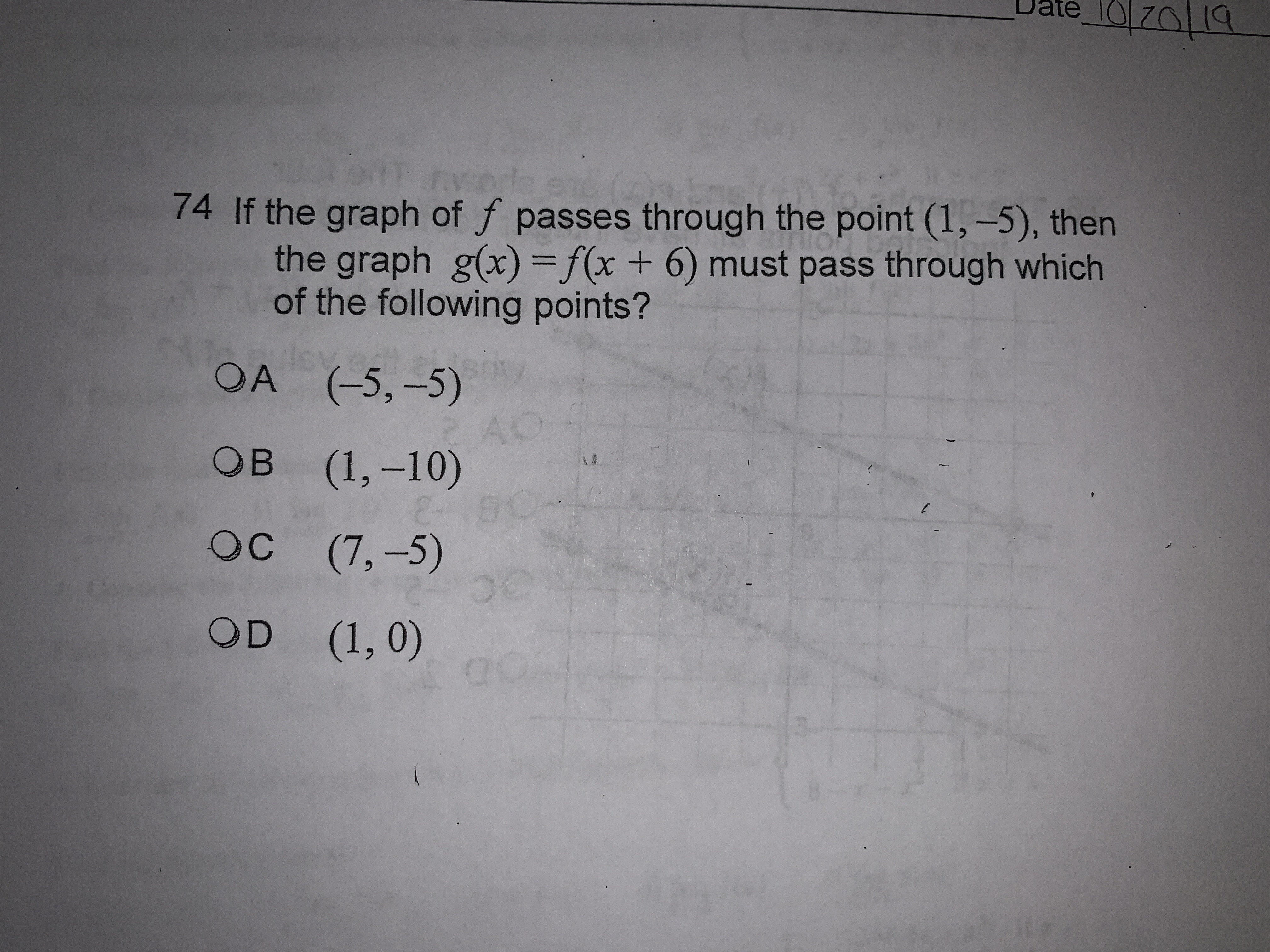# Date z074 If the graph of f passes th rough the point (1,-5), thenthe graph g(x) = f(x + 6) must pass through whichof the following points?OA (-5,-5)ACоB (1,-10)0ос (7,-5)OCOD (1,0)C

Question
30 views

if the graph f passes through point (1,-5) the graph g(x)=f(x+6) much pass through which of the following points?

A. (-5.-5)

B. (1,-10)

C. (7,-5)

D. (1,0)help_outlineImage TranscriptioncloseDate z0 74 If the graph of f passes th rough the point (1,-5), then the graph g(x) = f(x + 6) must pass through which of the following points? OA (-5,-5) AC оB (1,-10) 0 ос (7,-5) OC OD (1,0) C fullscreen
check_circle

star
star
star
star
star
1 Rating
Step 1

Since the graph f passes through point (1,-5), so we have

Step 2

Now, we set x+6=1 and find the value of x

Step 3

Now we find g(-5) by replacing x by...

### Want to see the full answer?

See Solution

#### Want to see this answer and more?

Solutions are written by subject experts who are available 24/7. Questions are typically answered within 1 hour.*

See Solution
*Response times may vary by subject and question.
Tagged in

### Other